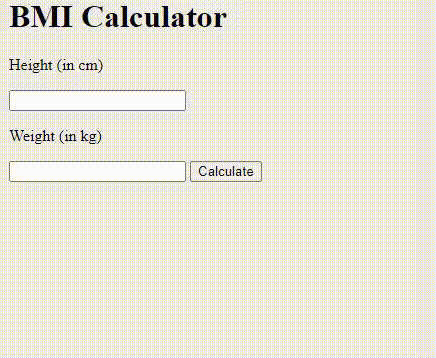Open in App
Not now

# Design a BMI Calculator using JavaScript

• Difficulty Level : Medium
• Last Updated : 11 Feb, 2021

The Body Mass Index (BMI) Calculator can be used to calculate BMI values based on their height and weight. BMI is a fairly reliable indicator of body fatness for most people.

Formula:

`BMI = (weight) / (height * height)`

Approach: BMI is a number calculated from an individual’s weight and height. To find out BMI we will take input from the user (both height and weight) which will be stored in height and weight variable for further calculation. The calculation process is simple, we will simply divide weight in kilograms by the square of the height. Now as per the BMI calculated, it will execute the respective if-else statement. We are also checking if the user is pressing submit button without entering the inputs, in that case, we are printing provide height or provide weight.
Using HTML we are giving desired structure, option for the input, and submit button. With the help of CSS, we are beautifying our structure by giving colors and desired font, etc.

In the JavaScript section, we are processing the taken input and after calculating, the respective output is printed.

Example:

HTML:

## index.html

 ` ` `<``html``> ` ` `  `<``head``> ` `    ``` `    ``<``script` `src``=``"app.js"``> ` ` ` ` `  `<``body``> ` `    ``<``div` `class``=``"container"``> ` `        ``<``h1``>BMI Calculator ` ` `  `        ``` `        ``<``p``>Height (in cm) ` ` `  `        ``<``input` `type``=``"text"` `id``=``"height"``> ` ` `  `        ``<``p``>Weight (in kg) ` ` `  `        ``<``input` `type``=``"text"` `id``=``"weight"``> ` ` `  `        ``<``button` `id``=``"btn"``>Calculate ` ` `  `        ``<``div` `id``=``"result"``> ` `    `` ` ` ` ` `  ` `

JavaScript:

## app.js

 `window.onload = () => { ` `    ``let button = document.querySelector(``"#btn"``); ` ` `  `    ``// Function for calculating BMI ` `    ``button.addEventListener(``"click"``, calculateBMI); ` `}; ` ` `  `function` `calculateBMI() { ` ` `  `    ``/* Getting input from user into height variable. ` `    ``Input is string so typecasting is necessary. */` `    ``let height = parseInt(document ` `            ``.querySelector(``"#height"``).value); ` ` `  `    ``/* Getting input from user into weight variable.  ` `    ``Input is string so typecasting is necessary.*/` `    ``let weight = parseInt(document ` `            ``.querySelector(``"#weight"``).value); ` ` `  `    ``let result = document.querySelector(``"#result"``); ` ` `  `    ``// Checking the user providing a proper ` `    ``// value or not ` `    ``if` `(height === ``""` `|| isNaN(height))  ` `        ``result.innerHTML = ``"Provide a valid Height!"``; ` ` `  `    ``else` `if` `(weight === ``""` `|| isNaN(weight))  ` `        ``result.innerHTML = ``"Provide a valid Weight!"``; ` ` `  `    ``// If both input is valid, calculate the bmi ` `    ``else` `{ ` ` `  `        ``// Fixing upto 2 decimal places ` `        ``let bmi = (weight / ((height * height)  ` `                            ``/ 10000)).toFixed(2); ` ` `  `        ``// Dividing as per the bmi conditions ` `        ``if` `(bmi < 18.6) result.innerHTML = ` `            ```Under Weight : \${bmi}`; ` ` `  `        ``else` `if` `(bmi >= 18.6 && bmi < 24.9)  ` `            ``result.innerHTML =  ` `                ```Normal : \${bmi}`; ` ` `  `        ``else` `result.innerHTML = ` `            ```Over Weight : \${bmi}`; ` `    ``} ` `} `

Output:My Personal Notes arrow_drop_up
Related Articles